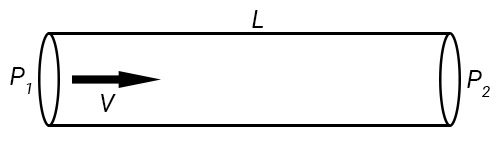# Darcy–Weisbach Equation Calculator

Created by Luis Hoyos
Last updated: Jul 09, 2022

Welcome to the Darcy–Weisbach equation calculator, a tool to calculate the pressure drop in a pipe. Input the pipe length, flow velocity, fluid density, friction factor, and pipe diameter, and the pressure drop will appear immediately.

• To calculate the pressure drop, you need to know the friction factor (f). You can find it using our darcy friction factor calculator.
• Use our flow rate calculator if you need to know the flow velocity but only know the flow rate.
• Density is another variable of the pressure drop equation. In our density calculator, you can find the density of many materials, including fluids.

Additionally, you can use this calculator in various ways, and not only to calculate the pressure drop. For example, if you're designing a pipe system, you can use it to know the necessary pipe diameter if pressure drop and flow conditions are known.

## Darcy–Weisbach equation for pressure drop

The equation to calculate pressure drop in a pipe is:

$\Delta P = f L ρ V^2/2D$

where:

• $\Delta P$ — Pressure drop, in Pascals (Pa);
• $f$ — Darcy friction factor, dimensionless;
• $L$ — Pipe length, in meters (m);
• $D$ — Pipe hydraulic diameter (pipe inner diameter), in meters (m);
• $\rho$ — Fluid density, in kg/m3; and
• $V$ — Average flow velocity through the pipe, in meters per second (m/s).

If you don't know the flow velocity, you can calculate it by remembering that flow velocity equals flow rate divided by the area covered by the hydraulic diameter:

$V = Q/A = Q/(πD^2/4) = 4Q/ \pi D^2$

where:

• $Q$ — Volumetric flow rate, in cubic meters per second (m³/s);
• $A$ — Area covered by the hydraulic diameter, in square meters ().

## Darcy friction factor calculation for pressure drop

To calculate the Darcy–Weisbach equation, calculating the Darcy friction factor is necessary. The friction factor formula will differ depending on whether the flow is laminar or turbulent.

### Friction factor for laminar flow

For laminar flow, the friction factor only depends on the dimensionless quantity known as Reynolds number (Re):

$f = 64/Re$

### Friction factor for turbulent flow

For turbulent flow, the friction factor is given by the Colebrook-White equation:

$\frac{1}{\sqrt{f}} = -2 \log{\left(\frac{k/D}{3.7} + \frac{2.52}{Re\sqrt{f}}\right)}$

where:

• $k$ — Pipe's surface roughness, in meters (m);
• $D$ — Pipe hydraulic diameter, in meters (m); and

The ratio $k/D$ is commonly known as relative roughness.

### Reynolds number

• We say a flow is laminar if its Reynolds number is below 2100 (Re < 2100).
• We consider a flow as turbulent if its Reynolds number exceeds 3000 (Re > 3000).
• When 2100 < Re < 3000, the flow is transitional, a situation in which it intermittently changes from laminar to turbulent.

For a pipe, the Reynolds number is given by:

$Re = ρVL/ \mu = VL/\nu$

where:

• $\mu$Dynamic viscosity of the fluid, in kg/(m·s); and
• $\nu$Kinematic viscosity of the fluid ($\nu = \mu/\rho$), in m²/s.

Hopefully, now you have a broader sense of the formulas behind this pipe pressure drop calculation. Thanks for reading!

Luis HoyosPipe length (L)
ft
Pipe diameter (D)
ft
Flow velocity (V)
ft/s
Density of fluid (ρ)
lb/cu ft
Darcy friction factor (f)
Pressure drop (ΔP)
psi
To calculate Darcy friction factor (f), try our dedicated friction factor calculator.
People also viewed…

### Coefficient of discharge

Use our coefficient of discharge calculator to find out the difference between theoretical and actual discharge rates.

### Flow rate

The flow rate calculator offers the estimation of volumetric and mass flow rates for different shapes of pipes.